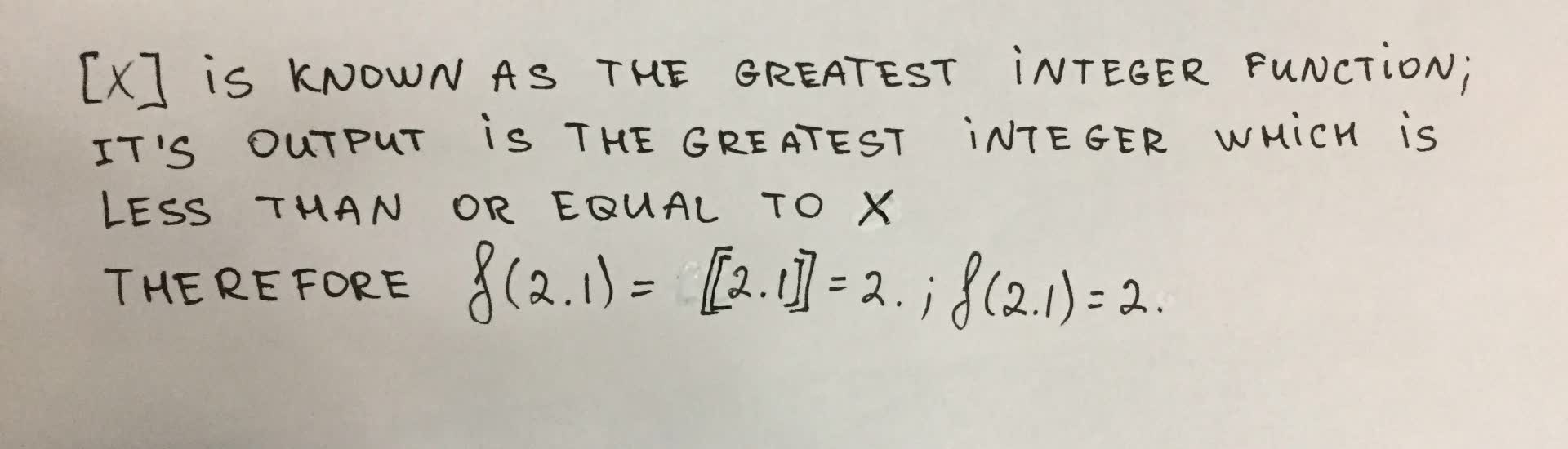# evaluate the function for the indicated values. f(x) = [x]. f(2.1)Chesley 2021-09-11 Answered
evaluate the function for the indicated values. $$\displaystyle{f{{\left({x}\right)}}}={\left[{x}\right]}.{f{{\left({2.1}\right)}}}$$

• Questions are typically answered in as fast as 30 minutes

### Plainmath recommends

• Get a detailed answer even on the hardest topics.
• Ask an expert for a step-by-step guidance to learn to do it yourself.faldduE

[x] is known as the greatest integer function. its output is the greatest integer which is less than or equal to x. Therefore $$\displaystyle{f{{\left({2.1}\right)}}}={\left[{\left[{2.1}\right]}\right]}={2}.{f{{\left({2.1}\right)}}}={2}$$